Get instant live expert help with Excel or Google Sheets“My Excelchat expert helped me in less than 20 minutes, saving me what would have been 5 hours of work!”

#### Post your problem and you'll get expert help in seconds

Your message must be at least 40 characters
Our professional experts are available now. Your privacy is guaranteed.

# Last row number in range

While working with Excel, we are able to determine the last row number of any data set by using the ROW and ROWS functions. There are also other formulas that use either the MIN or INDEX function in combination with ROW and ROWS.  This step by step tutorial will assist all levels of Excel users in determining the last row number in a range using three different formulas.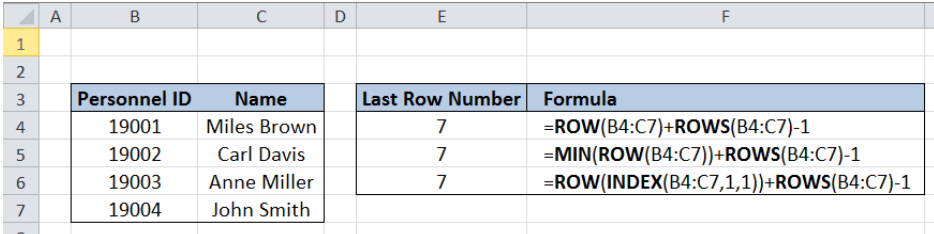Figure 1. Final result: Last row number in range

Formula 1:  `=ROW(B4:C7)+ROWS(B4:C7)-1`

Formula 2:  `=MIN(ROW(B4:C7))+ROWS(B4:C7)-1`

Formula 3:  `=ROW(INDEX(B4:C7,1,1))+ROWS(B4:C7)-1`

## Syntax of the ROW function

ROW function returns the row number of a reference

`=ROW(reference)`

• reference – The cell or range of cells whose row number we want to determine

## Syntax of the ROWS function

ROWS function returns the number of rows in a data set

`=ROW(array)`

• array– an array, range or reference for which we want to determine the number of rows

## Syntax of the MIN function

The MIN function returns the lowest value in a data set

`=MIN(number1, [number2], ...)  `

The parameters are:

• number1, number2, … – the numbers for which we want to find the lowest value; only number1 is required; succeeding numbers are optional
• The arguments could be numbers, array or reference to cells containing numbers

## Syntax of the INDEX function

The INDEX function returns a value as specified from within a range

`=INDEX(array, row_num, column_num)`

The parameters are:

• array – a range of cells where we want to retrieve some data
• row_num – the row in the array from which we want to retrieve data
• column_num – the column in the array from which we want to retrieve data

## Setting up Our Data

Our table contains a list of Personnel ID (column B) and Name (column C).  In cell E4, we want to determine the last row number of our range.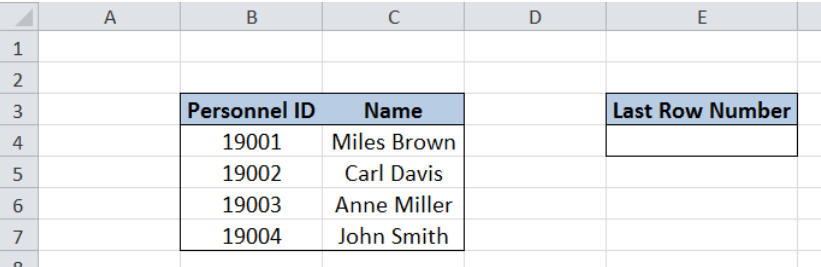Figure 2. Sample data for last row number in range

## Last row number using ROW and ROWS

The simplest formula to determine the last row number in a range is by using the ROW and ROWS functions.  We will follow these steps:

Step 1.  Select cell E4

Step 2.  Enter the formula: `=ROW(B4:C7)+ROWS(B4:C7)-1`

Step 3:  Press ENTER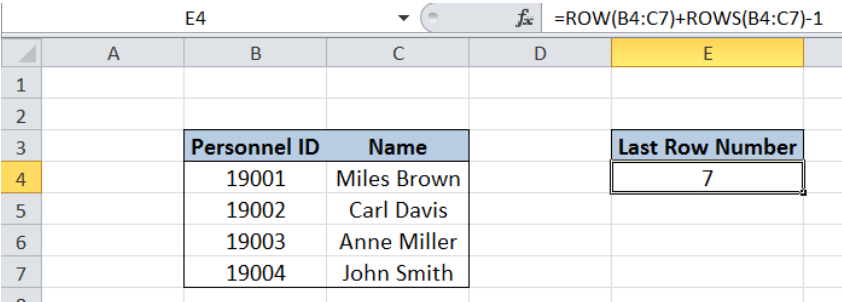Figure 3. Entering the formula using ROW and ROWS

The ROW function returns an array result of the row numbers in our range {4;5;6;7}.  However, when entered in a single cell, the ROW function returns only the first row in our range, which is 4.  The ROWS function also returns 4, because there are four rows in our range.  The formula becomes 4 + 4 – 1 = 7.

Therefore, the final result in cell E4 is 7, which is the last row number in our range.

## Last row number using MIN, ROW and ROWS

There is another formula that determines the last row number in a range is by using the MIN function in combination with ROW and ROWS.  Let us follow these steps:

Step 1.  Select cell E4

Step 2.  Enter the formula: `=MIN(ROW(B4:C7))+ROWS(B4:C7)-1`

Step 3:  Press ENTER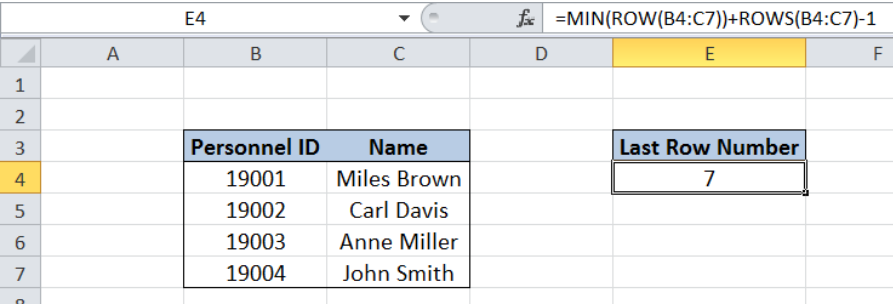Figure 4. Entering the formula using MIN, ROW and ROWS

As in the previous example, the ROW function returns an array result {4;5;6;7}. The MIN function returns the lowest value in the array, which is 4.  The ROWS function also returns 4.  The formula becomes 4 + 4 – 1 = 7.

Therefore, the final result in cell E4 is 7, which is the same as in the first formula using only ROW and ROWS.

## Last row number using INDEX, ROW and ROWS

There is a third formula that determines the last row number in a range is by using the INDEX function in combination with ROW and ROWS.  Let us follow these steps:

Step 1.  Select cell E4

Step 2.  Enter the formula: `=ROW(INDEX(B4:C7,1,1))+ROWS(B4:C7)-1`

Step 3:  Press ENTER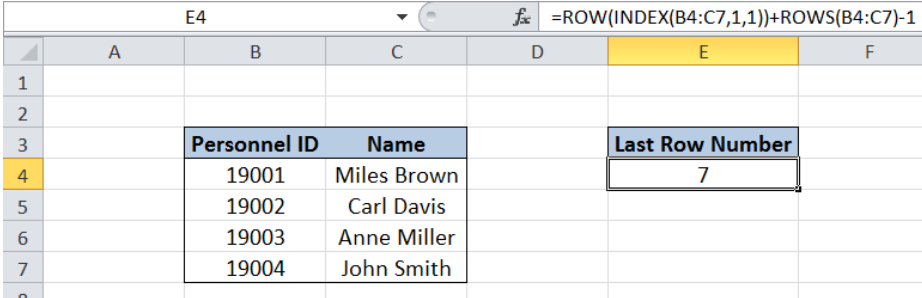Figure 5. Entering the formula using INDEX, ROW and ROWS

The INDEX function returns the first cell in our range, which is B4.  The ROW function returns 4, which is the row number of B4.  The ROWS function also returns 4. The formula becomes 4 + 4 – 1 = 7.

Finally, the result in cell E4 is also 7, which is the same as in the two previous examples.

The table below shows the three formulas having the same final result.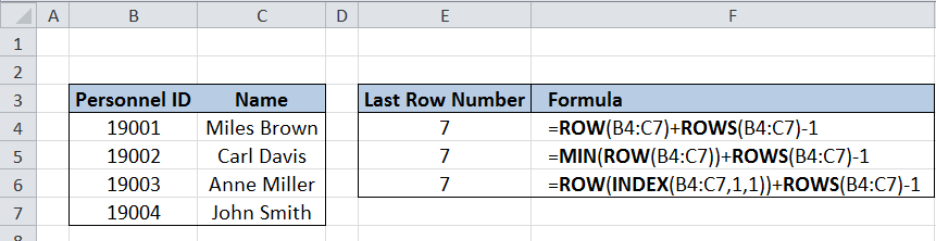Figure 6. Three different formulas to get the last row number in range

Most of the time, the problem you will need to solve will be more complex than a simple application of a formula or function. If you want to save hours of research and frustration, try our live Excelchat service! Our Excel Experts are available 24/7 to answer any Excel question you may have. We guarantee a connection within 30 seconds and a customized solution within 20 minutes.

### Did this post not answer your question? Get a solution from connecting with the expert.Another blog reader asked this question today on Excelchat:## Subscribe to Excelchat.coAnother blog reader asked this question today on Excelchat: